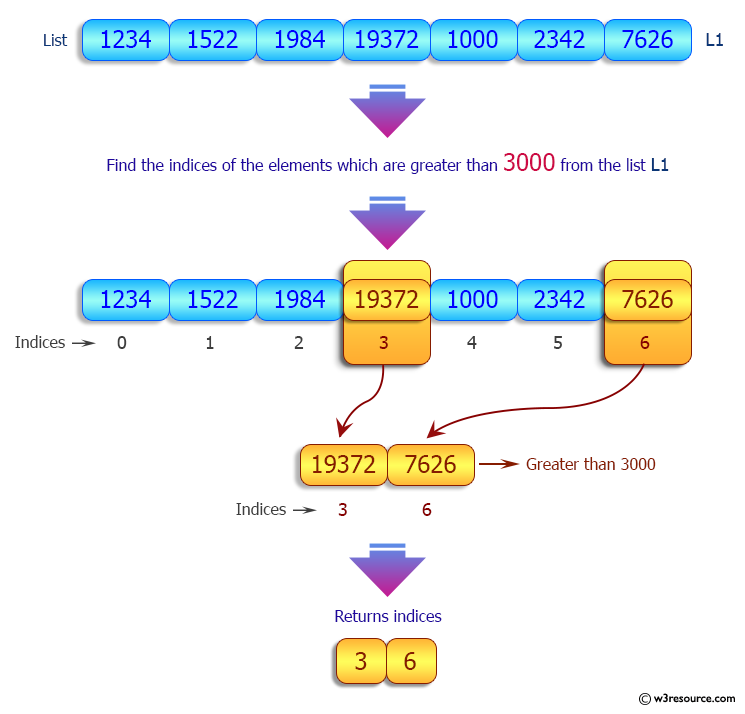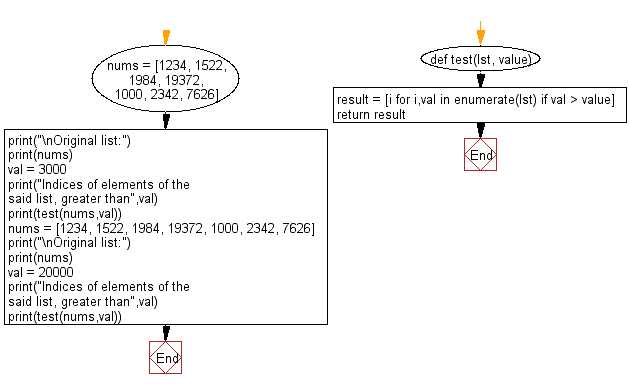﻿ Python: Find the indices of elements of a given list, greater than a specified value - w3resource

# Python: Find the indices of elements of a given list, greater than a specified value

## Python List: Exercise - 205 with Solution

Write a Python program to find the indices of elements in a given list that are greater than a specified value.

Sample Solution:

Python Code:

``````def test(lst, value):
result = [i for i,val in enumerate(lst) if val > value]
return result
nums = [1234, 1522, 1984, 19372, 1000, 2342, 7626]
print("\nOriginal list:")
print(nums)
val = 3000
print("Indices of elements of the said list, greater than",val)
print(test(nums,val))
nums = [1234, 1522, 1984, 19372, 1000, 2342, 7626]
print("\nOriginal list:")
print(nums)
val = 20000
print("Indices of elements of the said list, greater than",val)
print(test(nums,val))
```
```

Sample Output:

```Original list:
[1234, 1522, 1984, 19372, 1000, 2342, 7626]
Indices of elements of the said list, greater than 3000
[3, 6]

Original list:
[1234, 1522, 1984, 19372, 1000, 2342, 7626]
Indices of elements of the said list, greater than 20000
[]
```

Pictorial Presentation:Flowchart:## Visualize Python code execution:

The following tool visualize what the computer is doing step-by-step as it executes the said program:

Python Code Editor:

Have another way to solve this solution? Contribute your code (and comments) through Disqus.

What is the difficulty level of this exercise?

Test your Programming skills with w3resource's quiz.

﻿

## Python: Tips of the Day

Decapitalizes the first letter of a string:

Example:

```def tips_decapitalize(s, upper_rest=False):
return s[:1].lower() + (s[1:].upper() if upper_rest else s[1:])
print(tips_decapitalize('PythonTips'))
print(tips_decapitalize('PythonTips', True))
```

Output:

```pythonTips
pYTHONTIPS
```

We are closing our Disqus commenting system for some maintenanace issues. You may write to us at reach[at]yahoo[dot]com or visit us at Facebook